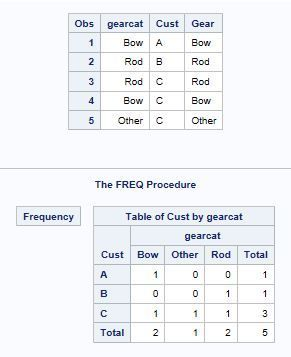## Proc Freq order=formatted not working (SAS EG (8.3.3.181) (64-bit))

I have followed Rick Wicklin's post about how to get Proc Freq to create tables in the order you want.  However, even though the data get sorted correctly, the output table is not displayed/sorted correctly.  Notice how when I printed the sorted dataset Cust "C" has all three gear types sorted correctly (Rod, Bow, Other); however, the output table using that variable (gearcat) and order=formatted, does not (instead output is alphabet).

My guess is this is because the first table value (Cust A) does not have all combinations of the second table variable (gearcat). I also tried using the "sparse" option in hopes that might help, but nothing changed.

My question: how can I get Proc Freq to use the sorted order of the second variable if/when the first entry of the first variable doesn't contain a full compliment of the second variable?  I tried a hack where I gave every customer every gear, but with zero weights for those not in the "real" data, but that didn't help.

``````/* Listing of Customers and Gears */

data test;
input Cust \$ Gear \$ ;
datalines;
A Bow
B Rod
C Bow
C Rod
C Other

;
run;

/* Create format that links Gear Categories in order to their labels */

proc format;
value gr
1='Rod'
2='Bow'
3='Other'
;
run;

/* Create numeric GearCat for sorting */
data test;
format gearcat gr.;
set test;
select (Gear);
when ('Rod') gearcat=1;
when ('Bow') gearcat=2;
when ('Other') gearcat=3;
when (' ') gearcat=.;
end;

/* Sort by Customer, then gearcat */

proc sort data=test; by cust gearcat;run;

proc print data=test;run; /* Shows the internal order is correct, see Customer C */

/* Create a 2-way table using order=formatted*/
/* Note how the order is wrong, even though the values were sorted properly */
/* Gearcat should be Rod - Bow - Other, not alphabetic */

proc freq data=test order=formatted;
tables cust*gearcat/nopercent nocol norow;
run;``````1 ACCEPTED SOLUTION

Accepted Solutions

## Re: Proc Freq order=formatted not working (SAS EG (8.3.3.181) (64-bit))

Since you replaced the text values with integers, just order the GEARCAT variable by the internal values 1,2,3. No need to sort:

``````
proc freq data=test order=internal;
tables cust*gearcat/nopercent nocol norow;
run;``````
6 REPLIES 6

## Re: Proc Freq order=formatted not working (SAS EG (8.3.3.181) (64-bit))

Since you replaced the text values with integers, just order the GEARCAT variable by the internal values 1,2,3. No need to sort:

``````
proc freq data=test order=internal;
tables cust*gearcat/nopercent nocol norow;
run;``````

## Re: Proc Freq order=formatted not working (SAS EG (8.3.3.181) (64-bit))

Thanks!

Still curious why the "formatted" option did not work, but pleased this does.

## Re: Proc Freq order=formatted not working (SAS EG (8.3.3.181) (64-bit))

For gearcat, the raw values are 1,2,3 and the corresponding formatted values are {'Rod' 'Bow' 'Others'}. If you sort by the formatted values, you will get the order 'Bow' 'Others' 'Rod'.

## Re: Proc Freq order=formatted not working (SAS EG (8.3.3.181) (64-bit))

Thanks. Between you and Ballardw I now better understand how my misunderstanding of "order=formatted" was causing my confusion.

## Re: Proc Freq order=formatted not working (SAS EG (8.3.3.181) (64-bit))

Partially a misunderstanding of the interaction between "formatted"  and the values you have specifically chosen. In FORMATTED order "Other" comes before "Rod" and "Bow" comes before "Other" because that is the sort order of the text values.

Your Sort doesn't use the formatted value of Gearcat, it uses the numeric value, so the formatted values have nothing to do with the Sort order displayed by Proc Print.

You may want to consider looking at this with another procedure that allows more Order options:

```proc tabulate data=test;
class gearcat /order=formatted;
format gearcat gr.;
class cust;
table gearcat,
cust
;
run;

proc tabulate data=test;
class gearcat /order=data;
format gearcat gr.;
class cust;
table gearcat,
cust
;
run;

proc tabulate data=test;
class gearcat /order=unformatted;
format gearcat gr.;
class cust;
table gearcat,
cust
;
run;
```

And for more fun, try changing your sort order around and then using this

```proc tabulate data=test;
format gearcat gr.;
class cust;
table gearcat,
cust
/printmiss
;
run;
```

In fact, you might try several sort orders with the previous.

## Re: Proc Freq order=formatted not working (SAS EG (8.3.3.181) (64-bit))

Indeed. My misunderstanding of what it meant when I asked for "order = formatted".

Thanks!
Discussion stats
• 6 replies
• 745 views
• 4 likes
• 3 in conversation